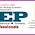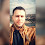Hazen Williams Excel Sheet Calculator for Head Loss Calculations

# Hazen Williams Excel Sheet Calculator for Head Loss Calculations

The Hazen–Williams equation describes the relationship which relates the flow of water in a pipe with the physical properties of the pipe. It can be used to calculate the pressure drop (psi) or friction loss in pipes or tubes.Hazen Williams Excel Sheet Calculator

Hazen–Williams Calculator excel sheet is a simple tool for obtaining the total head loss in a pipe by entering these parameters:

• Pipe material (or enter the C value instead)
• Inside diameter
• Flow rate
• Pipe length
• Fluid velocity

The software includes solved examples for:
• Hazen williams equation
• Table 1. Pipe inner diameter of various pipe specifications (Uni-Bell, 2001)
• Table 2. Equivalent pipe length of common fittings (CIDWT, 2008)

1.Thanks a lot Mr. Ahmad, I appreciate.

1.you are welcome

2.We need videos how to use these software and sheets pls

.

3.Pls upload videos how to use these software and Excel sheets that will very helpful
Thanks